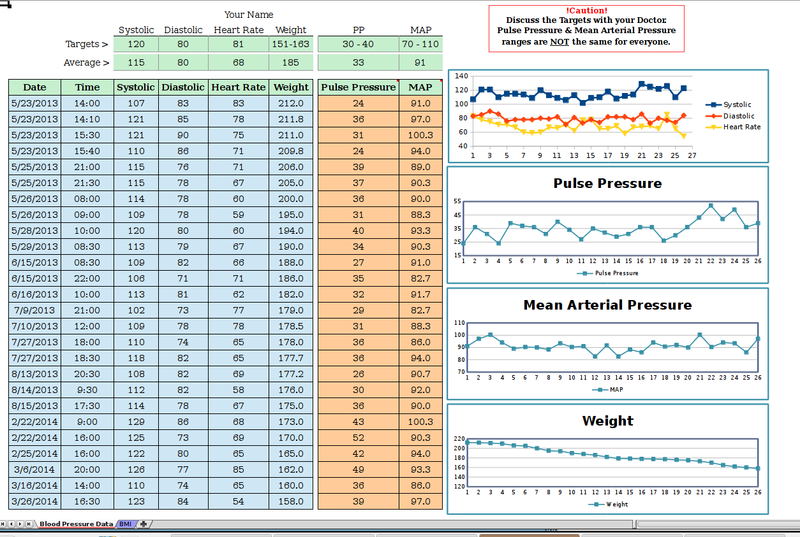# Blood Pressure - Weight - BMI

A spreadsheet to track blood pressure, weight and body mass index.## Description

Please consult your doctor prior to beginning any exercise or weight loss program. This sheet allows one to track Blood Pressure, Heart Rate, Weight,

Pulse Pressure, Mean Arterial Pressure and Body Mass Index. The sheet calculates Averages for Systolic, Diastolic, Heart Rate, Weight, Pulse Pressure, (PP), and Mean Arterial Pressure, (MAP).

These cells contain formulas so make a back up copy prior to changing things.

The formula for MAP is taken from wikipedia and can be reviewed at the link below.

The only data entry on the BMI tab is your height in inches. Example, 5ft,9in would be (12 x 5) + 9 = 69 inches.

The BMI formula explanation: "The BMI for a person is defined as their body mass divided by the square of their height—with the value universally being given in units of kg/m2. So if the weight is in kilograms and the height in meters, the result is immediate, if pounds and inches are used, a conversion factor of 703 (kg/m2)/(lb/in2) must be applied."

Source: https://en.wikipedia.org/wiki/Body_mass_index

https://en.wikipedia.org/wiki/Mean_arterial_pressure

https://en.wikipedia.org/wiki/Pulse_pressure

https://en.wikipedia.org/wiki/Body_mass_index

The Project Resources links do not work.

Copy & Paste the link below for the template. - Oct 14, 2018

https://github.com/hd625b/BP_BMI